Math resources Geometry Surface area

Surface area of a pyramid

# Surface area of a pyramid

Here you will learn about the surface area of a pyramid, including what it is and how to calculate it.

Students will first learn about the surface area of a pyramid as part of geometry in 6th grade.

## What is the surface area of a pyramid?

The surface area of a pyramid is the total sum of all the faces of the pyramid. A pyramid is a 3D shape made up of a polygonal base and triangular lateral faces. Pyramids are named after the shape of their bases.

For example,

A square pyramid consists of a square base and four triangular faces:

Square pyramidNetComponent faces

The triangular faces appear to have the same height. When the vertex of a pyramid is directly above the center of the base, the slant height (or the height of each triangular face) is the same. When the vertex is not directly above, the slant heights of the lateral sides will vary.

A rectangular pyramid consists of a rectangle base, and four triangular faces:

Rectangular pyramidNetComponent faces

A triangular pyramid consists of a triangle base, and three triangular faces:

Triangular pyramidNetComponent faces

To calculate the surface area of a pyramid, calculate the area of each face of the pyramid and then add the areas together.

For example,

All lateral faces of this square pyramid are congruent. Find the surface area.

\text{Area of the base }=5\times{5}=25\text{ cm}^{2}

\text{Area of a triangular face }=\cfrac{1}{2}\times{8}\times{5}=20\text{ cm}^{2}

Add the area of the base and the 4 congruent triangular faces:

\text{Surface area }=25+20+20+20+20=25+(4\times{20})=105\text{ cm}^{2}

The total surface area can also be written in one equation:

\begin{aligned} \text{Surface area of pyramid }&= \text{ Area of base } + \text{ Area of triangular faces} \\\\ &=5^2+4 \times (\cfrac{1}{2} \, \times 5 \times 8) \\\\ &=25+80 \\\\ &=105 \; cm^2 \end{aligned}

### What is the surface area of a pyramid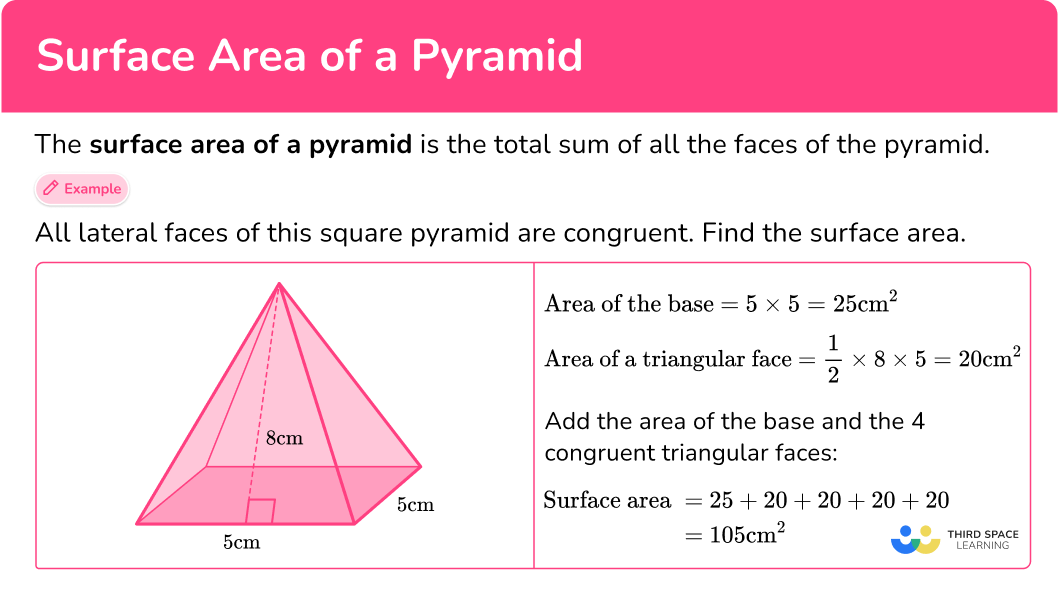## Common Core State Standards

How does this relate to 6th grade math?

• Grade 6 – Geometry (6.G.A.4)
Represent three-dimensional figures using nets made up of rectangles and triangles, and use the nets to find the surface area of these figures. Apply these techniques in the context of solving real-world and mathematical problems.

## How to find the surface area of a pyramid

In order to find the surface area of a pyramid:

1. Calculate the area of each face.
2. Add the area of each face together.
3. Include the units.

## Surface area of a pyramid examples

### Example 1: square-based pyramid in cm

All the lateral faces of the pyramid are congruent. Calculate the surface area.

1. Calculate the area of each face.

The base is a square with the area 6\times{6}=36\text{ cm}^2.

All four triangular faces are identical, so calculate the area of one triangle and then multiply the area by 4.

\begin{aligned} A&=\cfrac{1}{2} \, \times{b}\times{h}\\\\ &=\cfrac{1}{2} \, \times{10}\times{6}\\\\ &=30 \end{aligned}

30\times{4}=120

2Add the area of each face together.

Add the area of the base and the area of the four triangles:

SA=36+120=156

3Include the units.

The side lengths are measured in centimeters, so the area is measured in square centimeters.

SA=156\text{ cm}^{2}

### Example 2: surface area of a triangular pyramid – net

The net of a triangular pyramid is shown below. All the lateral faces of the pyramid are congruent. Calculate the surface area.

The area of the triangular base is \cfrac{1}{2} \, \times 8.1 \times 7=28.35.

All three lateral triangular faces are identical, so calculate the area of one triangle and then multiply the area by 3 .

\begin{aligned} & A=\cfrac{1}{2} \, \times b \times h \\\\ & =\cfrac{1}{2} \, \times 8.1 \times 14.3 \\\\ & =57.915 \end{aligned}

57.915 \times 3=173.745

Add the area of the base and the area of the four triangles:

S A=28.35+173.745=202.095

The side lengths are measured in inches, so the area is measured in square inches.

S A=202.095 \text { inches }^2

### Example 3: surface area of a square pyramid

Above is a square pyramid where all lateral faces have the same slant height. The perimeter of the base is 4m . Calculate the total surface area of the square pyramid.

Since the base is a square, each side length of the base is the same. To find one side length, divide the base perimeter by 4 :

4 \div 4=1 \mathrm{~m}

Each side of the base is 1 \times 1=1 m^2.

Each side of the face is a congruent isosceles triangle.

\begin{aligned} A&=\cfrac{1}{2} \, \times{b}\times{h} \\\\ &=\cfrac{1}{2} \, \times{1}\times{1.2}\\\\ &=0.6\text{ m}^{2} \end{aligned}

4\times{0.6}=2.4\text{ m}^2

Add the area of the base and the area of the four triangles:

SA=1+2.4=3.4

SA=3.4\text{ m}^{2}

### Example 4: rectangular-based pyramid – lateral surface area

ABCDE is a rectangular-based pyramid. The opposite lateral faces are congruent. Calculate the lateral surface area of the pyramid.

The units for the base are not the same, so convert all the units to meters.

90 \mathrm{~cm}=0.9 \mathrm{~m}

Since you are finding the lateral surface area of the pyramid, you do not need to calculate the area of the base.

The pyramid has two pairs of congruent faces:

\text { Triangle } A D E=\text { Triangle } B C E

and

\text { Triangle } C D E=\text { Triangle } A B E

The area of Triangle ADE is:

A=\cfrac{1}{2} \times 1.2 \times 3.8=2.28

\text { Triangle } B C E is congruent to \text { Triangle } A D E, so its area is also 2.28.

The area of \text { Triangle } C D E is:

A=\cfrac{1}{2} \times 0.9 \times 3.82=1.719

\text { Triangle } A B E is congruent to \text { Triangle } C D E , so its area is also 1.719.

Adding the area of all of the lateral faces together:

\begin{aligned} L S A&=2.28+2.28+1.719+1.719 \\\\ & =2(2.28)+2(1.719) \\\\ & =4.56+3.438 \\\\ & =7.998 \end{aligned}

L S A=7.998 \, m^2

### Example 5: pyramid – right triangles

In the triangle above, the slant height from vertex D to side \overline{C A} is 7.8 , so the height for \text{triangle CDA} is 7.8 . Calculate the surface area of the triangle.

The base is the right triangle CBA . The area is \cfrac{1}{2} \, \times 7 \times 7=24.5 .

There are 3 lateral triangular faces. Two of them, \text { triangle } C B D and \text { triangle } D B A , are congruent.

The area of right triangles CBD and DBA is \cfrac{1}{2} \, \times 7 \times 6=21.

The other lateral face is the triangle CDA .

The base is 9.9 and the height is 7.8 , so the area of the triangle is \cfrac{1}{2} \, \times 9.9 \times 7.8=38.61.

Add the area of the base and the area of the three triangles:

S A=24.5+21+21+38.61=105.11

In this case, no units are given, so the surface area can be labeled with general units squared.

S A=105.11 \text { units}^2

### Example 6: rectangular-based pyramid – fractions

Calculate the surface area of the pyramid.

Remember that the edges in a pyramid are always equal, so if you were to fold up the net, the 3 \cfrac{1}{5} \mathrm{~cm} side of the triangle and 4 \cfrac{3}{4} \mathrm{~cm} side will combine to form an edge with each corresponding side – making their lengths equal.

The opposite sides of the rectangle are also equal.

The area of the base is:

\begin{aligned} A & =3 \cfrac{1}{2} \, \times 2 \cfrac{2}{3} \\\\ & =\cfrac{7}{2} \, \times \frac{8}{3} \\\\ & =\cfrac{56}{6} \\\\ & =9 \cfrac{2}{6} \, \text { or } \, 9 \frac{1}{3} \end{aligned}

The area of the far left triangle is:

\begin{aligned} A & =\cfrac{1}{2} \, \times 2 \, \cfrac{2}{3} \, \times 3 \cfrac{1}{5} \\\\ & =\cfrac{1}{2} \, \times \cfrac{8}{3} \, \times \cfrac{16}{5} \\\\ & =\cfrac{128}{30} \\\\ & =4 \cfrac{8}{30} \, \text { or } \, 4 \cfrac{4}{15} \end{aligned}

The area of the top triangle is:

\begin{aligned} A & =\cfrac{1}{2} \, \times 3 \, \cfrac{1}{2} \, \times 3 \, \cfrac{1}{5} \\\\ & =\cfrac{1}{2} \, \times \, \cfrac{7}{2} \, \times \, \cfrac{16}{5} \\\\ & =\cfrac{112}{20} \\\\ & =5 \, \cfrac{12}{20} \, \text { or } \, 5 \frac{3}{5} \end{aligned}

The area of the far right triangle is:

\begin{aligned} A & =\cfrac{1}{2} \, \times 2 \, \cfrac{2}{3} \, \times 4 \, \cfrac{3}{4} \\\\ & =\cfrac{1}{2} \, \times \, \cfrac{8}{3} \, \times \, \cfrac{19}{4} \\\\ & =\cfrac{152}{24} \\\\ & =6 \, \cfrac{8}{24} \, \text { or } \, 6 \frac{1}{3} \end{aligned}

The area of the bottom triangle is:

\begin{aligned} A & =\cfrac{1}{2} \, \times 3 \, \cfrac{1}{2} \, \times 4 \, \cfrac{1}{5} \\\\ & =\cfrac{1}{2} \, \times \, \cfrac{7}{2} \, \times \, \cfrac{21}{5} \\\\ & =\cfrac{147}{20} \\\\ & =7 \, \cfrac{7}{20} \end{aligned}

Add the area of the base and the area of the four triangles:

\begin{aligned} S A & =9 \cfrac{1}{3}+4 \cfrac{4}{15}+6 \cfrac{1}{3}+7 \cfrac{7}{20} \\\\ & =9 \cfrac{20}{60}+4 \cfrac{16}{60}+6 \cfrac{20}{60}+7 \cfrac{21}{60} \\\\ & =26 \cfrac{77}{60} \\\\ & =27 \cfrac{17}{60} \end{aligned}

S A=27 \,\cfrac{17}{60} \mathrm{~cm}^2

### Teaching tips for the surface area of a pyramids

• Give students plenty of opportunities to work with physical 3D models and nets before asking them to solve with surface area.

• Choose worksheets that provide a variety of pyramids – a mixture of triangle, square and rectangles as bases; a mixture of congruent and non-congruent lateral faces; a mixture of pyramid drawings, nets or word problems given.

• Include projects where students have the opportunity to create nets from 3D objects.

### Easy mistakes to make

• Thinking the side of a triangle is always the height
The height of the triangle needs to be the perpendicular height. Only if the side is perpendicular to the base can it be used as the height.

• Thinking surface area is units or units cubed
The surface area will have square units such as square centimeters \mathrm{cm}^2 or square meters \mathrm{m}^2 . Regular units are for one-dimensional measurements like length or height, and cubed units are used for measuring volume.

• Thinking opposite lateral faces are always equal
This is only true when the apex (top vertex) is directly over the center of the base. While this is often the type of pyramids that are given, it is not always the case. Instead of assuming, always use the information given.

### Practice surface area of a pyramid questions

1. All the lateral faces of the pyramid are congruent. Calculate the surface area of the pyramid.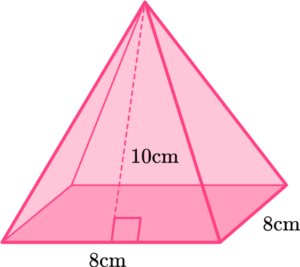384 \text{ cm}^2260 \text{ cm}^2140 \text{ cm}^2224 \text{ cm}^2\begin{aligned} \text{Surface area of pyramid }&= \text{Area of base} + \text{Area of triangular faces}\\\\ &=8^2+4\times (\cfrac{1}{2} \, \times 8\times 10)\\\\ &=64+160\\\\ &=224 \ cm^2 \end{aligned}

2. The pyramid is composed of congruent equilateral triangles. Find the surface area of the pyramid.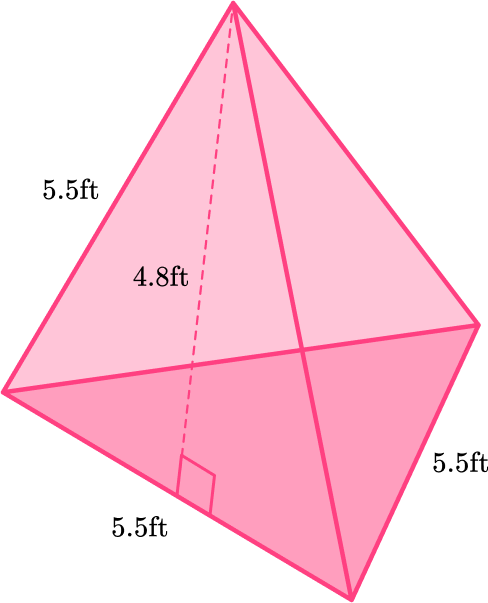69.85 \mathrm{~ft}^260.5 \mathrm{~ft}^252.8 \mathrm{~ft}^254.725 \mathrm{~ft}^2\begin{aligned} \text { Surface area of pyramid }&= \text { Area of base and faces } \\ & \quad \text{ (} 4 \text { congruent triangles) } \\\\ & =4 \times\left(\cfrac{1}{2} \, \times 5.5 \times 4.8\right) \\\\ & =4 \times 13.2 \\\\ & =52.8 \mathrm{~ft}^2 \end{aligned}

3. The opposite lateral faces of ABCD are congruent. Calculate the surface area of the pyramid.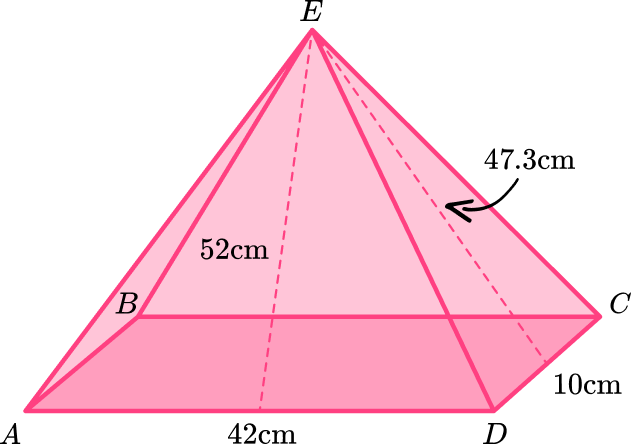3,077 \mathrm{~cm}^27,280 \mathrm{~cm}^24,788 \mathrm{~cm}^21,366 \mathrm{~cm}^2\begin{aligned} \text{Surface area of pyramid}&= \text{Area of base} + \text{Area of triangular faces}\\\\ = \, (&42 \times{10})+2\times (\cfrac{1}{2} \, \times{42}\times{52})+2\times (\cfrac{1}{2} \, \times{10}\times{47.3})\\\\ = 4&20+2,184+473\\\\ = 3&,077 \text{ cm}^2 \end{aligned}

4. Here is the net of a square-based pyramid with congruent lateral faces. Calculate the surface area of the lateral faces: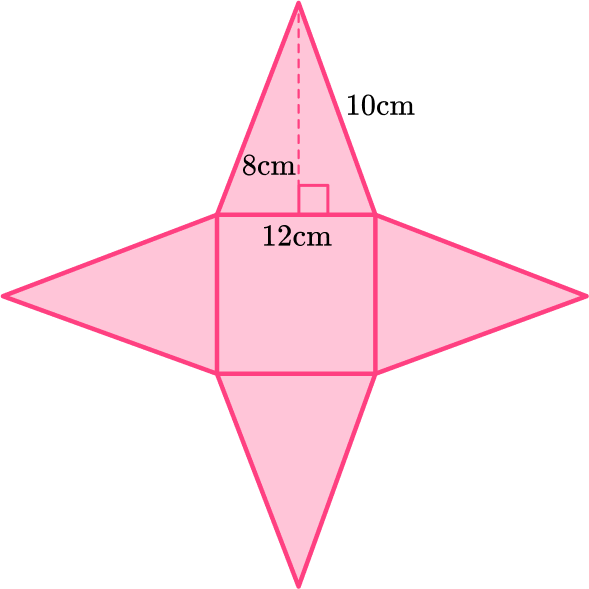240 \text{ cm}^2384 \text{ cm}^2192 \text{ cm}^2336 \text{ cm}^2\begin{aligned} \text { Lateral surface area of pyramid } & =\text { Areas of triangular faces } \\\\ & =4 \times\left(\cfrac{1}{2} \times 12 \times 8\right) \\\\ & =4 \times 48 \\\\ & =192 \mathrm{~cm}^2 \end{aligned}

5. Below is a square pyramid with congruent lateral faces. The perimeter of the base is 12.4 \, cm. Calculate the surface area to the nearest whole number.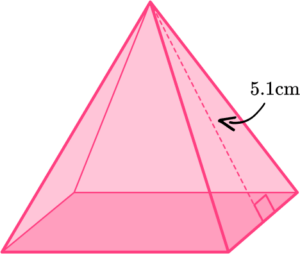16 \mathrm{~cm}^241 \mathrm{~cm}^217 \mathrm{~cm}^242\ cm^2One side of the square can be found by dividing the perimeter by 4 :

12.4 \div 4=3.1 \mathrm{~cm}.

\begin{aligned} \text{Surface area of pyramid }&=\text{Area of base }+ \text{Areas of triangular faces} \\\\ & =3.1^2+4 \times \left(\cfrac{1}{2} \, \times 3.1 \times 5.1\right) \\\\ & =9.61+31.62 \\\\ & =41.23 \end{aligned}

Rounded to the nearest whole, the surface area is 41 \, cm^2.

6. Here is the net of a square-based pyramid. Calculate the surface area of the pyramid.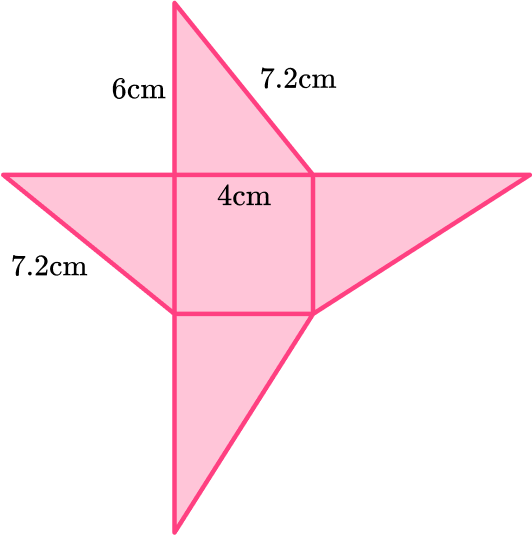48 \mathrm{~cm}^2112 \mathrm{~cm}^264 \mathrm{~cm}^268.8 \mathrm{~cm}^2Remember that the edges in a pyramid are always equal, so if you were to fold up the net, the 6 \, cm side of the triangle and 7.2 \, cm side will combine to form an edge with each corresponding side – making their lengths equal.

All sides of the square are also equal.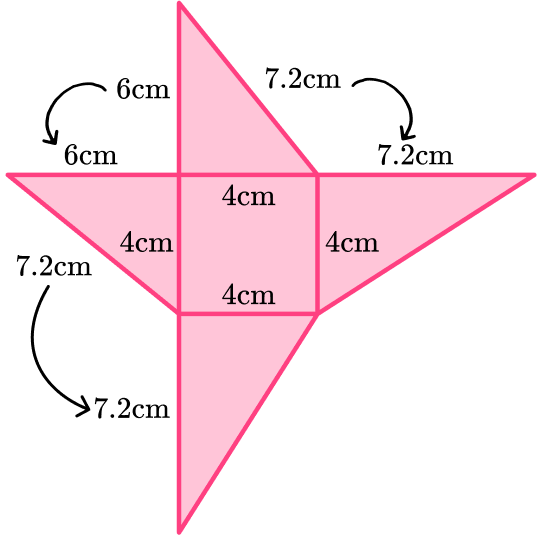The area of the base is 4\times{4}=16\text{ cm}^2

The area of two of the triangles is:

\left(\cfrac{1}{2} \, \times{6}\times{4}\right)\times{2}=24\text{ cm}^2

The area of the other two triangles is:

\left(\cfrac{1}{2} \, \times 4 \times 7.2\right) \times 2=28.8 \mathrm{~cm}^2

The total surface area is therefore:

S A=16+24+28.8=68.8 \mathrm{~cm}^2

## Surface area of a pyramid FAQs

Is there a surface area of a triangular pyramid formula?

Because the types of triangles in a pyramid vary, the formula for the surface area is very general. S A=\text { Area of base }+ \text { Area of } 3 \text { lateral faces } .

There are pentagonal pyramids that have a pentagon as a base. There are hexagonal pyramids that have a hexagon as a base. These are just two examples – there are many more. The different types of pyramids are named after the polygonal shape of their base.

What is a regular pyramid?

A pyramid whose base is a regular polygon and whose lateral faces are the same height. The surface area of a regular pyramid always involves congruent lateral faces.

## Still stuck?

At Third Space Learning, we specialize in helping teachers and school leaders to provide personalized math support for more of their students through high-quality, online one-on-one math tutoring delivered by subject experts.

Each week, our tutors support thousands of students who are at risk of not meeting their grade-level expectations, and help accelerate their progress and boost their confidence.